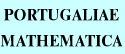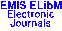PORTUGALIAEMATHEMATICA Vol. 58, No. 4, pp. 485-504 (2001)

Previous Article

Contents of this Issue

Other Issues

ELibM Journals

ELibM Home

EMIS Home

## Micro-Support and Cauchy Problem for Temperate Solutions of Regular $\cal D$-Modules

### Masaki Kashiwara, Teresa Monteiro Fernandes and Pierre Schapira

Research Institute for Mathematical Sciences, Kyoto University,
Kyoto 606-8502 -- JAPAN
E-mail: masaki@kurims.kyoto-u.ac.jp
Centro de Álgebra da Universidade de Lisboa, Complexo 2,
2, Avenida Prof. Gama Pinto, 1699 Lisboa codex -- PORTUGAL
E-mail: tmf@ptmat.lmc.fc.ul.pt
Université Pierre et Marie Curie, case 82, Analyse Algébrique, UMR7586,
4, place Jussieu,75252 Paris cedex 05 -- FRANCE
E-mail: schapira@math.jussieu.fr
http://www.math.jussieu.fr/ schapira/

Abstract: Let $X$ be a complex manifold, $V$ a smooth involutive submanifold of $T^*X$, $\cal M$ a microdifferential system regular along $V$, and $F$ an $\R$-constructible sheaf on $X$. We study the complex of temperate microfunction solutions of $\cal M$ associated with $F$, that is, the complex $R\Hom_{{\cal D}_X}({\cal M},{\cal T}\mu\hom(F,{\cal O}_X))$. We give a bound to its micro-support and solve the Cauchy problem under a suitable hyperbolicity assumption.

Full text of the article: